# ICSE Class 8 Maths Selina Solutions Chapter 8 Profit Loss and Discount

Profit and loss play an important role in running businesses. Students must have heard that company A made a profit of 50 Lakhs in the year 2018. But do they know how the profit and loss are being calculated? For this students have to study Chapter 8 of ICSE Class 8 which deals with the Profit Loss and Discount. In this chapter, students will find questions related to daily life problems.

The experts at BYJU’S have provided the answers in pdf format for ICSE Class 8 Maths Selina Solutions Chapter 8 Profit Loss and Discount. The questions are solved in the easiest way so that students can understand the solutions. To download the pdf click on the link below;

## Download ICSE Class 8 Maths Selina Solutions PDF for Chapter 8:-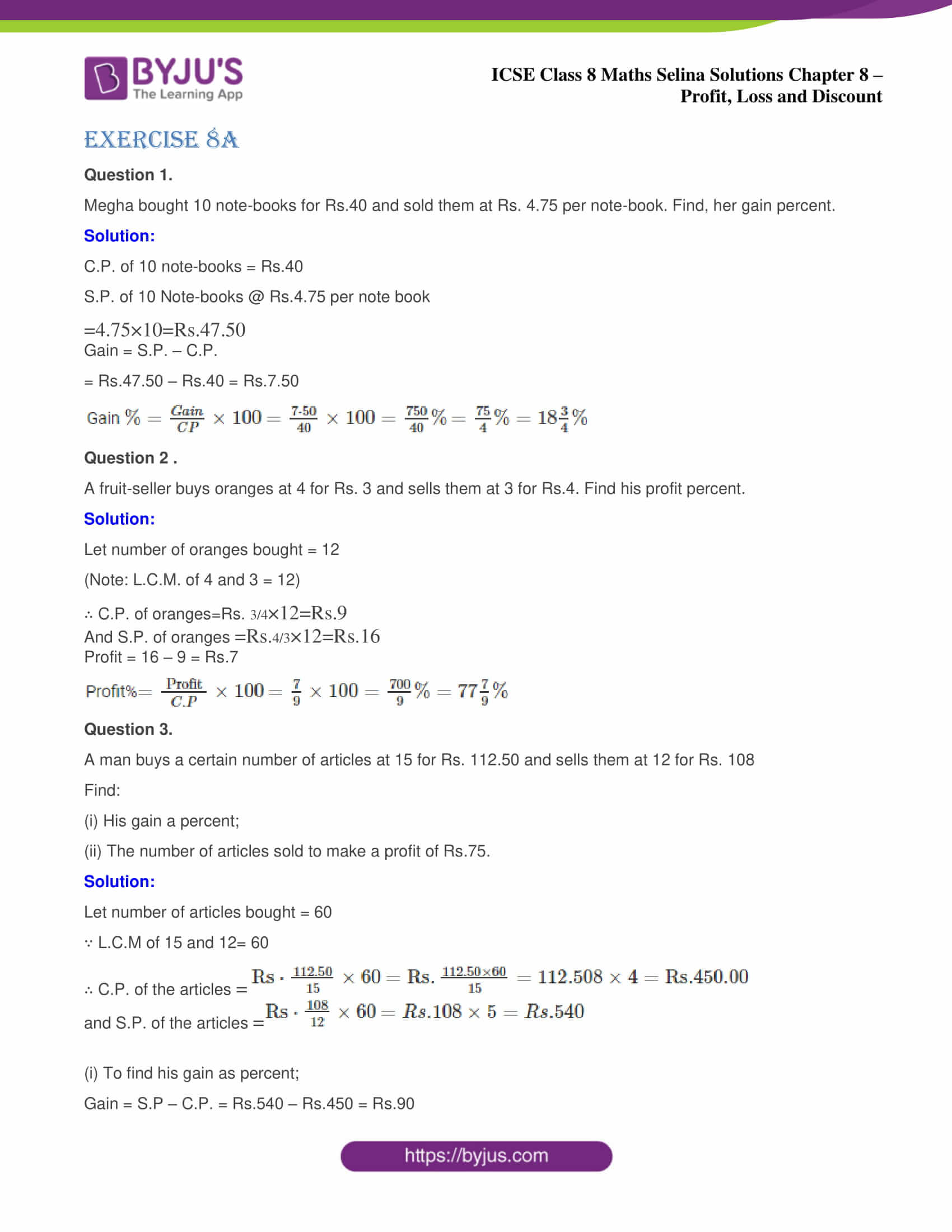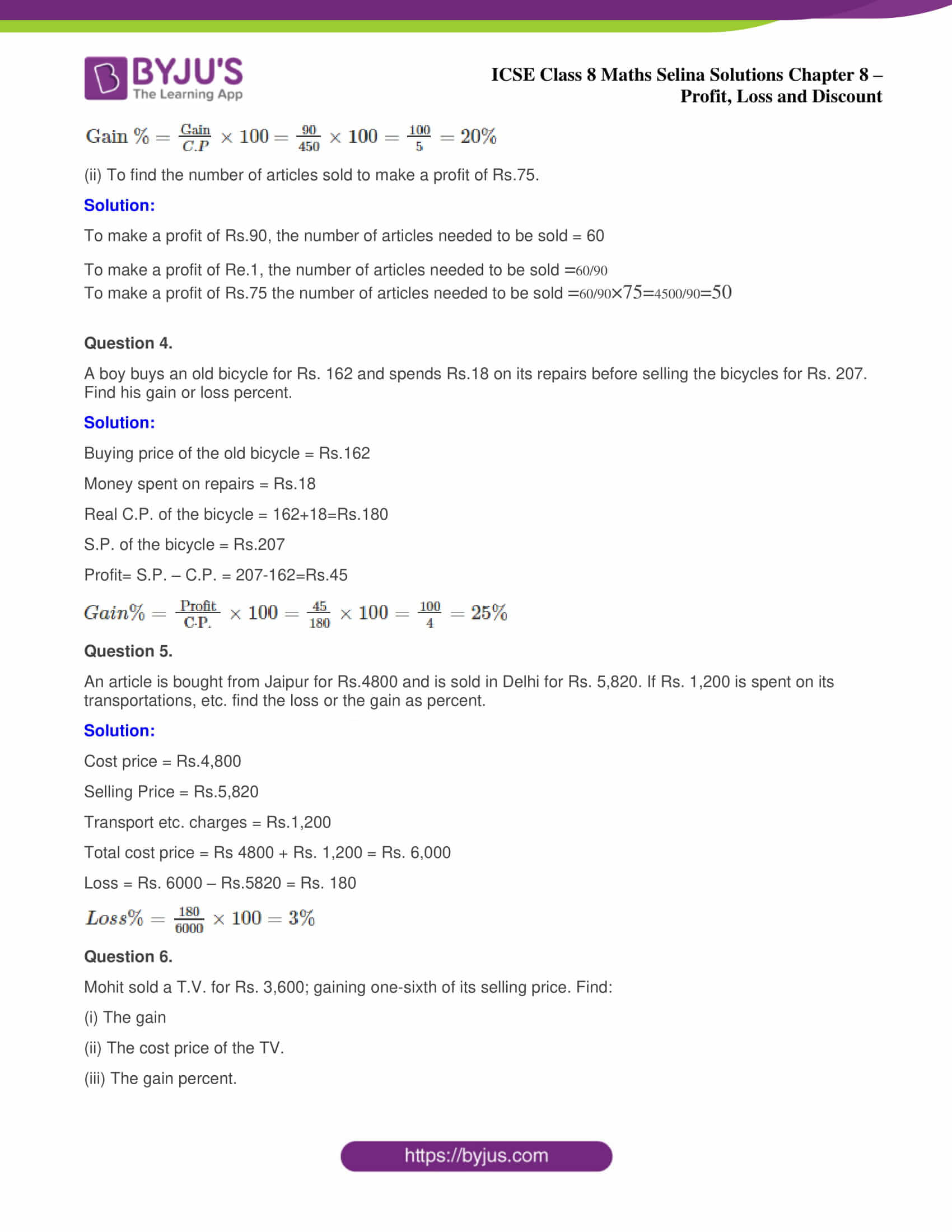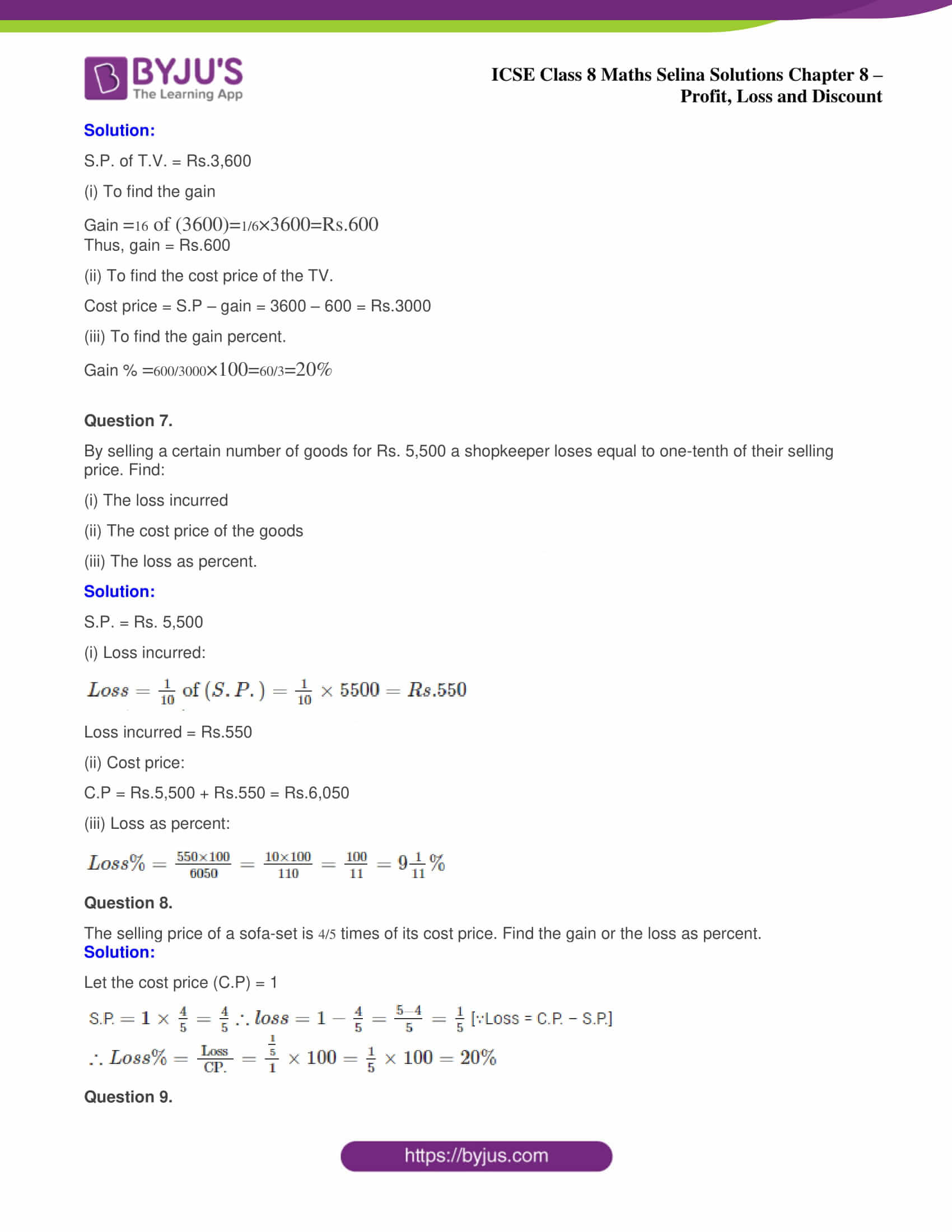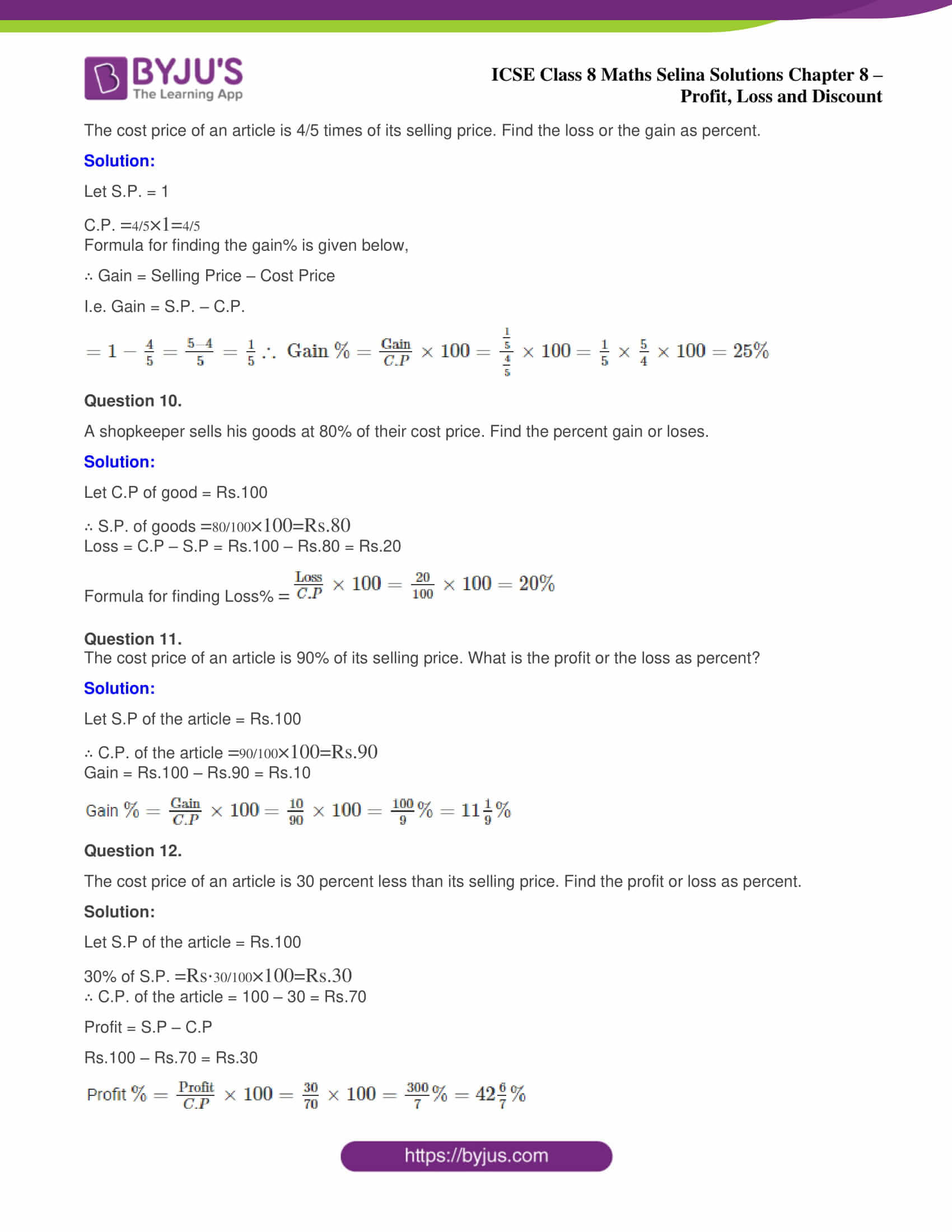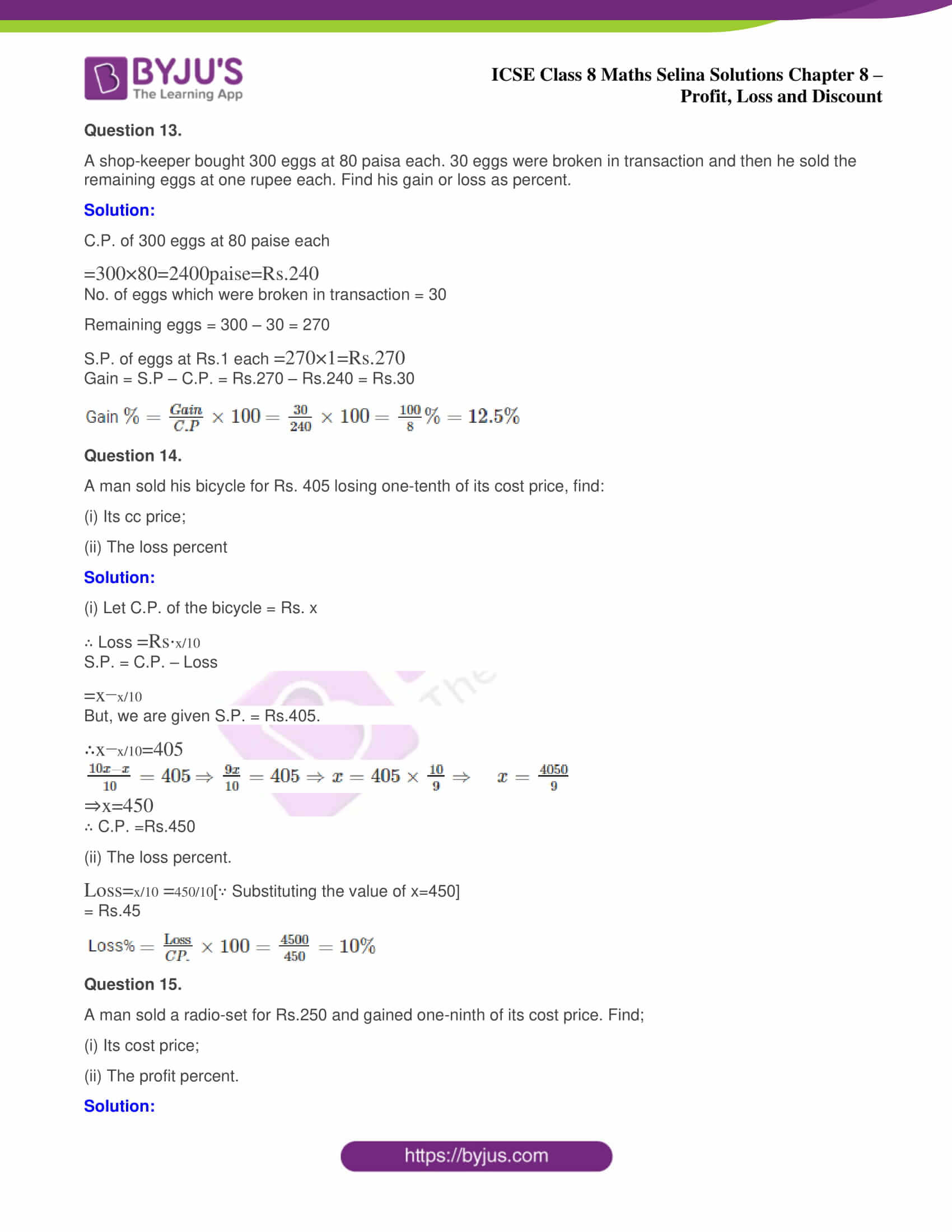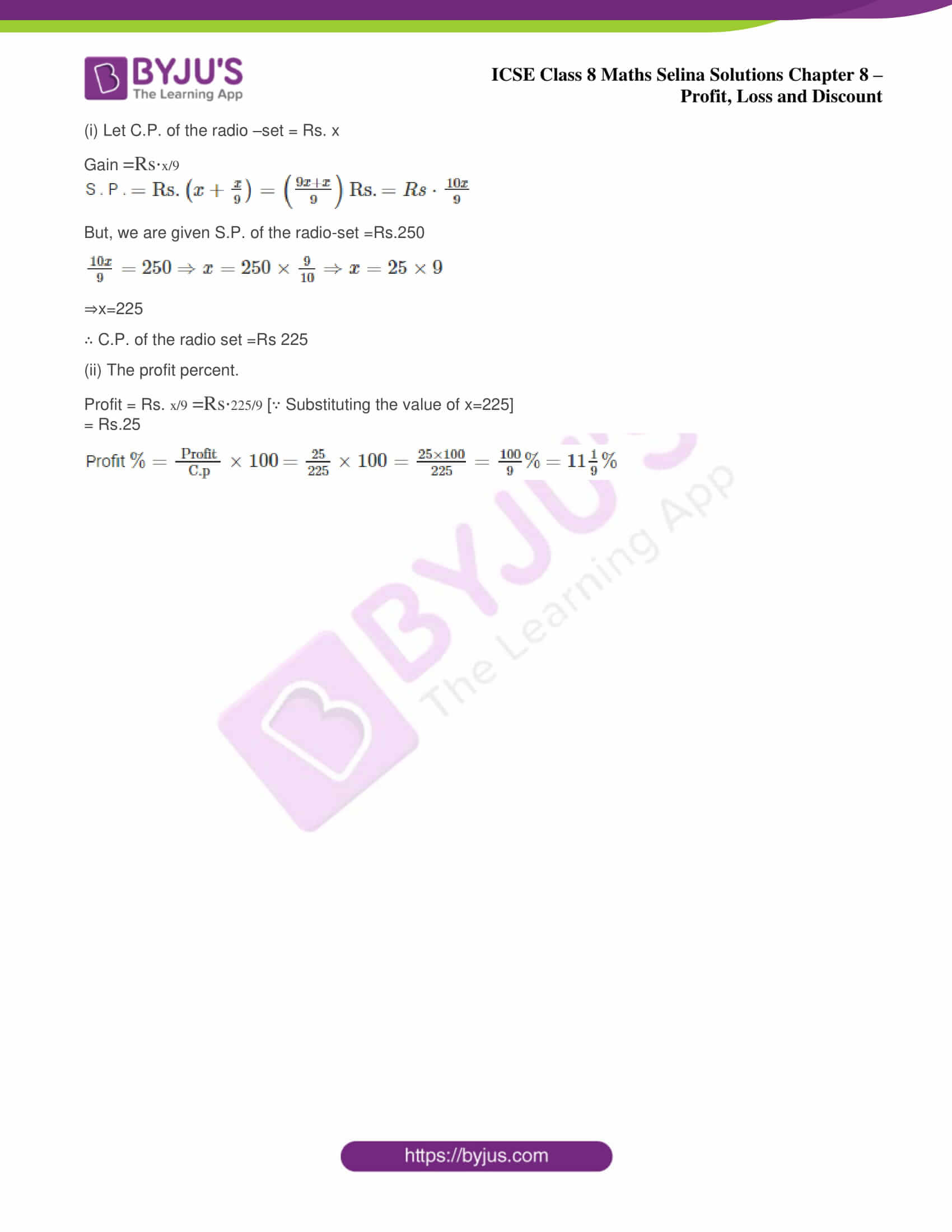ICSE Class 8 Maths Chapter 8 – Profit Loss and Discount has only one exercise i.e 8 (A). It contains a total of 14 questions. The step by step solutions to all these questions are also provided below:

### ICSE Class 8 Maths Selina Solutions Chapter 8 Profit Loss and Discount – Exercise 8 (A)

Question 1.

Megha bought 10 note-books for Rs.40 and sold them at Rs. 4.75 per note-book. Find, her gain percent.

Solution:

C.P. of 10 note-books = Rs.40

S.P. of 10 Note-books @ Rs.4.75 per note book

$$\begin{array}{l} =4.75 \times 10=\mathrm{Rs} .47 .50\end{array}$$

Gain = S.P. – C.P.

= Rs.47.50 – Rs.40 = Rs.7.50

Gain

$$\begin{array}{l} \%=\frac{{Gain}}{C P} \times 100\end{array}$$
$$\begin{array}{l} =\frac{7 \cdot 50}{40} \times 100=\frac{750}{40} \%\end{array}$$
$$\begin{array}{l} =\frac{75}{4} \%=18 \frac{3}{4} \%\end{array}$$

Question 2 .

A fruit-seller buys oranges at 4 for Rs. 3 and sells them at 3 for Rs.4. Find his profit percent.

Solution:

Let number of oranges bought = 12

(Note: L.C.M. of 4 and 3 = 12)

∴ C.P. of oranges=Rs.

$$\begin{array}{l} \frac{3}{4} \times 12=\mathrm{Rs} .9\end{array}$$

And S.P. of oranges

$$\begin{array}{l} = Rs. \frac{4}{3} \times 12=R s .16\end{array}$$

Profit = 16 – 9 = Rs.7

Profit%

$$\begin{array}{l} =\frac{\text { Profit }}{C . P} \times 100\end{array}$$
$$\begin{array}{l} =\frac{7}{9} \times 100=\frac{700}{9} \%=77 \frac{7}{9} \%\end{array}$$

Question 3.

A man buys a certain number of articles at 15 for Rs. 112.50 and sells them at 12 for Rs. 108

Find:

(i) His gain a percent;

(ii) The number of articles sold to make a profit of Rs.75.

Solution:

Let number of articles bought = 60

∵ L.C.M of 15 and 12= 60

∴ C.P. of the articles

$$\begin{array}{l} =\mathrm{Rs} \cdot \frac{112.50}{15} \times 60\end{array}$$
$$\begin{array}{l} =\mathrm{Rs} . \frac{112.50 \times 60}{15}=112.508 \times 4=\mathrm{Rs} .450 .00\end{array}$$

and S.P. of the articles

$$\begin{array}{l} =\mathrm{Rs} \cdot \frac{108}{12} \times 60\end{array}$$
$$\begin{array}{l} =R s .108 \times 5=R s .540\end{array}$$

(i) To find his gain as percent;

Gain = S.P – C.P. = Rs.540 – Rs.450 = Rs.90

$$\begin{array}{l} ∴ \quad \text { Gain } \%=\frac{\text {Gain}}{C . P} \times 100\end{array}$$
$$\begin{array}{l} =\frac{90}{450} \times 100=\frac{100}{5}=20 \%\end{array}$$

(ii) To find the number of articles sold to make a profit of Rs.75.

Solution:

To make a profit of Rs.90, the number of articles needed to be sold = 60

To make a profit of Re.1, the number of articles needed to be sold

$$\begin{array}{l} =\frac{60}{90}\end{array}$$

To make a profit of Rs.75 the number of articles needed to be sold

$$\begin{array}{l} =\frac{60}{90} \times 75=\frac{4500}{90}=50 \end{array}$$

Question 4.

A boy buys an old bicycle for Rs. 162 and spends Rs.18 on its repairs before selling the bicycles for Rs. 207. Find his gain or loss percent.

Solution:

Buying price of the old bicycle = Rs.162

Money spent on repairs = Rs.18

Real C.P. of the bicycle = 162+18=Rs.180

S.P. of the bicycle = Rs.207

Profit= S.P. – C.P. = 207-162=Rs.45

$$\begin{array}{l} {Gain} \%=\frac{\text { Profit }}{\mathrm{C} \cdot \mathrm{P} .} \times 100=\frac{45}{180} \times 100=\frac{100}{4}=25 \%\end{array}$$

Question 5.

An article is bought from Jaipur for Rs.4800 and is sold in Delhi for Rs. 5,820. If Rs. 1,200 is spent on its transportations, etc. find the loss or the gain as percent.

Solution:

Cost price = Rs.4,800

Selling Price = Rs.5,820

Transport etc. charges = Rs.1,200

Total cost price = Rs 4800 + Rs. 1,200 = Rs. 6,000

Loss = Rs. 6000 – Rs.5820 = Rs. 180

$$\begin{array}{l} ∴ {Loss} \%=\frac{180}{6000} \times 100=3 \%\end{array}$$

Question 6.

Mohit sold a T.V. for Rs. 3,600; gaining one-sixth of its selling price. Find:

(i) The gain

(ii) The cost price of the TV.

(iii) The gain percent.

Solution:

S.P. of T.V. = Rs.3,600

(i) To find the gain

Gain

$$\begin{array}{l} =\frac{1}{6} \text { of }(3600)=\frac{1}{6} \times 3600=\mathrm{Rs} .600\end{array}$$

Thus, gain = Rs.600

(ii) To find the cost price of the TV.

Cost price = S.P – gain = 3600 – 600 = Rs.3000

(iii) To find the gain percent.

Gain %

$$\begin{array}{l} =\frac{600}{3000} \times 100=\frac{60}{3}=20 \% \end{array}$$

Question 7.

By selling a certain number of goods for Rs. 5,500 a shopkeeper loses equal to one-tenth of their selling price. Find:

(i) The loss incurred

(ii) The cost price of the goods

(iii) The loss as percent.

Solution:

S.P. = Rs. 5,500

(i) Loss incurred:

$$\begin{array}{l} {Loss}=\frac{1}{10} \text { of }(S . P .)=\frac{1}{10} \times 5500=R s .550 ) \end{array}$$

Loss incurred = Rs.550

(ii) Cost price:

C.P = Rs.5,500 + Rs.550 = Rs.6,050

(iii) Loss as percent:

$$\begin{array}{l} {Loss} \%=\frac{550 \times 100}{6050}=\frac{10 \times 100}{110}=\frac{100}{11}=9 \frac{1}{11} \%\end{array}$$

Question 8.

The selling price of a sofa-set is

$$\begin{array}{l} \frac{4}{5}\end{array}$$
times of its cost price. Find the gain or the loss as percent.

Solution:

Let the cost price (C.P) = 1

S.P.

$$\begin{array}{l} =1 \times \frac{4}{5}=\frac{4}{5}\end{array}$$

$$\begin{array}{l} ∴ {loss} =1-\frac{4}{5}=\frac{5-4}{5}=\frac{1}{5}\end{array}$$
[∵Loss = C.P. – S.P.]

$$\begin{array}{l} ∴ {Loss} \%=\frac{\text { Loss }}{\mathrm{CP} .}=\frac{\frac{1}{5}}{1} \times 100=\frac{1}{5} \times 100=20 \%\end{array}$$

Question 9.

The cost price of an article is 4/5 times of its selling price. Find the loss or the gain as percent.

Solution:

Let S.P. = 1

C.P.

$$\begin{array}{l} =\frac{4}{5} \times 1=\frac{4}{5} \end{array}$$

Formula for finding the gain% is given below,

∴ Gain = Selling Price – Cost Price

I.e. Gain = S.P. – C.P.

$$\begin{array}{l} =1-\frac{4}{5}=\frac{5-4}{5}=\frac{1}{5}\end{array}$$

$$\begin{array}{l} \text { Gain } \%=\frac{\text {Gain}}{C . P} \times 100=\frac{\frac{1}{5}}{\frac{4}{5}} \times 100\end{array}$$
$$\begin{array}{l} =\frac{1}{5} \times \frac{5}{4} \times 100=25 \%\end{array}$$

Question 10.

A shopkeeper sells his goods at 80% of their cost price. Find the percent gain or loses.

Solution:

Let C.P of good = Rs.100

∴ S.P. of goods

$$\begin{array}{l} =\frac{80}{100} \times 100=R s .80\end{array}$$

Loss = C.P – S.P = Rs.100 – Rs.80 = Rs.20

Formula for finding Loss%

$$\begin{array}{l} =\frac{\text {Loss}}{C . P} \times 100=\frac{20}{100} \times 100=20 \%\end{array}$$

Question 11.

The cost price of an article is 90% of its selling price. What is the profit or the loss as percent?

Solution:

Let S.P of the article = Rs.100

∴ C.P. of the article

$$\begin{array}{l} =\frac{90}{100} \times 100=\mathrm{Rs} .90\end{array}$$

Gain = Rs.100 – Rs.90 = Rs.10

Gain

$$\begin{array}{l} \%=\frac{\text {Gain}}{C . P} \times 100\end{array}$$
$$\begin{array}{l} =\frac{10}{90} \times 100=\frac{100}{9} \%=11 \frac{1}{9} \%\end{array}$$

Question 12.

The cost price of an article is 30 percent less than its selling price. Find the profit or loss as percent.

Solution:

Let S.P of the article = Rs.100

30% of S.P.

$$\begin{array}{l} =\mathrm{Rs} \cdot \frac{30}{100} \times 100=R s .30\end{array}$$

∴ C.P. of the article = 100 – 30 = Rs.70

Profit = S.P – C.P

Rs.100 – Rs.70 = Rs.30

Profit

$$\begin{array}{l} \%=\frac{\text {Profit}}{C . P} \times 100=\frac{30}{70} \times 100=\frac{300}{7} \%=42 \frac{6}{7} \%\end{array}$$

Question 13.

A shop-keeper bought 300 eggs at 80 paisa each. 30 eggs were broken in transaction and then he sold the remaining eggs at one rupee each. Find his gain or loss as percent.

Solution:

C.P. of 300 eggs at 80 paise each

$$\begin{array}{l} =300 \times 80=2400 paise = Rs.240\end{array}$$

No. of eggs which were broken in transaction = 30

Remaining eggs = 300 – 30 = 270

S.P. of eggs at Rs.1 each

$$\begin{array}{l} =270 \times 1=\mathrm{Rs} .270\end{array}$$

Gain = S.P – C.P. = Rs.270 – Rs.240 = Rs.30

Gain

$$\begin{array}{l} \%=\frac{{Gain}}{C . P} \times 100\end{array}$$
$$\begin{array}{l} =\frac{30}{240} \times 100\end{array}$$
$$\begin{array}{l} =\frac{100}{8} \%=12.5 \%\end{array}$$

Question 14.

A man sold his bicycle for Rs. 405 losing one-tenth of its cost price, find:

(i) Its cc price;

(ii) The loss percent

Solution:

(i) Let C.P. of the bicycle = Rs. x

∴ Loss

$$\begin{array}{l} =\mathrm{Rs} \cdot \frac{x}{10}\end{array}$$

S.P. = C.P. – Loss

$$\begin{array}{l} =x-\frac{x}{10}\end{array}$$

But, we are given S.P. = Rs.405.

$$\begin{array}{l} ∴ \quad x-\frac{x}{10}=405 \end{array}$$
$$\begin{array}{l} \Rightarrow \frac{10 x-x}{10}=405\end{array}$$
$$\begin{array}{l} \Rightarrow \frac{9 x}{10}=405 \Rightarrow x=405 \times \frac{10}{9}\end{array}$$
$$\begin{array}{l} \Rightarrow \quad x=\frac{4050}{9} \Rightarrow x=450\end{array}$$

∴ C.P. =Rs.450

(ii) The loss percent.

$$\begin{array}{l} Loss =\frac{x}{10}\end{array}$$
$$\begin{array}{l} =\frac{450}{10} \end{array}$$
[∵ Substituting the value of x=450]

= Rs.45

Loss%

$$\begin{array}{l} =\frac{\text {Loss}}{C P .} \times 100=\frac{4500}{450}=10 \%\end{array}$$

Question 15.

A man sold a radio-set for Rs.250 and gained one-ninth of its cost price. Find;

(i) Its cost price;

(ii) The profit percent.

Solution:

(i) Let C.P. of the radio –set = Rs. x

Gain

$$\begin{array}{l} =\mathrm{Rs} \cdot \frac{x}{9}\end{array}$$

S . P .

$$\begin{array}{l} =\mathrm{Rs} .\left(x+\frac{x}{9}\right)=\left(\frac{9 x+x}{9}\right) \mathrm{Rs} .=R s \cdot \frac{10 x}{9}\end{array}$$

But, we are given S.P. of the radio-set =Rs.250

$$\begin{array}{l} ∴ \quad \frac{10 x}{9}=250\end{array}$$
$$\begin{array}{l} \Rightarrow x=250 \times \frac{9}{10} \Rightarrow x=25 \times 9 \Rightarrow x=225\end{array}$$

⇒x=225

∴ C.P. of the radio set =Rs225

(ii) The profit percent.

Profit = Rs.

$$\begin{array}{l} \frac{x}{9}\end{array}$$
$$\begin{array}{l} =\mathrm{Rs} \cdot \frac{225}{9}\end{array}$$
[∵ Substituting the value of x=225]

= Rs.25

Profit

$$\begin{array}{l} \%=\frac{\text { Profit }}{\text {C.p}} \times 100\end{array}$$
$$\begin{array}{l} =\frac{25}{225} \times 100=\frac{25 \times 100}{225}=\frac{100}{9} \% \end{array}$$
$$\begin{array}{l} =11 \frac{1}{9} \% \end{array}$$

To get the Selina Solutions for the chapters of class 8 Maths, visit the ICSE Class 8 Maths Selina Solutions page.

### ICSE Class 8 Maths Selina Solutions Chapter 8 – Profit Loss and Discount

The concepts learned in percentage will be used here when students have to solve the problems related to the profit or loss percentage. In this chapter, students will learn some new terms such as cost price, selling price, marked price, discount etc. Also, they will get to know the formulas for solving the problems related to them. Slightly advanced problems are also provided in the exercise for students brainstorming.

Also, students can get the answers of Maths, Physics, Chemistry and Biology subjects by clicking on ICSE Class 8 Selina Solutions.

We hope this information on “ICSE Class 8 Maths Selina Solutions Chapter 8 Profit Loss and Discount” is useful for students. Keep learning and stay tuned for further updates on ICSE and other competitive exams. To access interactive Maths and Science Videos download BYJU’S App and subscribe to YouTube Channel.